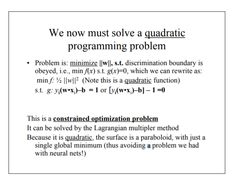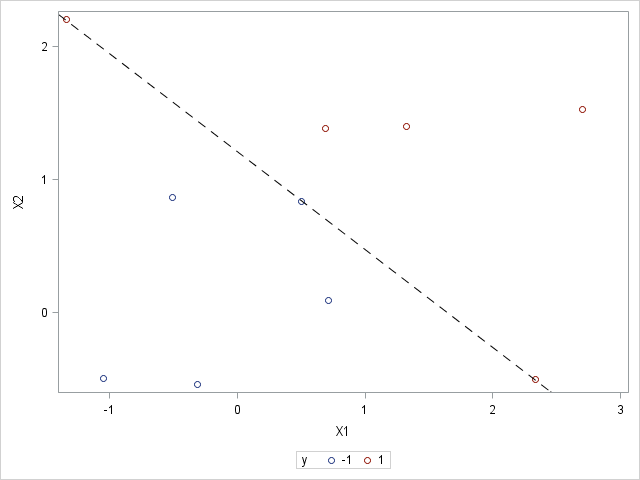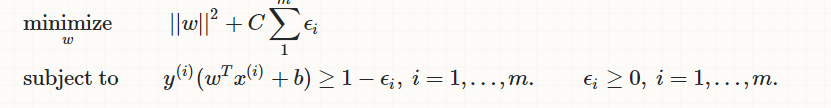## Is possible to apply SVM with proc optmodel?

Hi for all!

I'm seeing powerful of proc optmodel and i would like to resolve svm problem with it.  is it possible to resolve support vector machine with it? Like this problemSuppose we have two vars linearly separable, I know proc svm can do it.

1 ACCEPTED SOLUTION

Accepted Solutions

## Re: Is possible to apply SVM with proc optmodel?

Here's a simple way to do it.  I included plots because you have two-dimensional data.

``````%let n = 2;
data indata;
input X1 X2 y;
datalines;
0.49922066	0.834590569	-1
-0.509598752	0.865356638	-1
-0.313012311	-0.54000741	-1
0.713675823	0.094540165	-1
-1.05062909	-0.493905705	-1
1.326427551	1.400617167	1
0.688668461	1.385509561	1
2.705730511	1.526311825	1
2.335457445	-0.497797479	1
-1.340637901	2.205443013	1
;

proc sgplot data=indata;
scatter x=x1 y=x2 / group=y;
run;

proc optmodel;
set OBS;
num n = &n;
num x {OBS, 1..n}, y {OBS};
read data indata into OBS=[_N_] {j in 1..n} <x[_N_,j]=col('x'||j)> y;

/* declare optimization model */
var W {1..n};
var B;
min Z = 0.5 * sum {j in 1..n} W[j]^2;
con Separate {i in OBS}:
y[i] * (sum {j in 1..n} x[i,j]*W[j] - B) >= 1;

/* call QP solver */
solve;

/* plot output */
num slope = -W.sol/W.sol;
num intercept = B.sol/W.sol;
submit slope intercept;
proc sgplot data=indata;
scatter x=x1 y=x2 / group=y;
lineparm x=0 y=&intercept slope=&slope / lineattrs=(pattern=dash color=black);
run;
endsubmit;
quit;``````15 REPLIES 15

## Re: Is possible to apply SVM with proc optmodel?

PROC OPTMODEL doesn't has a SVM algorithm. It has transitive closure, shortest path, minimum cost network flow, minimum spanning tree, minimum cut, TSP, among others, but not SVM. For SVM you need to use Enterprise Miner or Viya VDMML.

## Re: Is possible to apply SVM with proc optmodel?

Although PROC OPTMODEL does not have anything built-in for SVM, you can formulate the quadratic programming (QP) problem and call the QP solver.

## Re: Is possible to apply SVM with proc optmodel?

Suposse we have this data:

``` X1	          X2            y
0.49922066	0.834590569	-1
-0.509598752	0.865356638	-1
-0.313012311	-0.54000741	-1
0.713675823	0.094540165	-1
-1.05062909	-0.493905705	-1
1.326427551	1.400617167	1
0.688668461	1.385509561	1
2.705730511	1.526311825	1
2.335457445	-0.497797479	1
-1.340637901	2.205443013	1```

how can we apply proc optmodel?

## Re: Is possible to apply SVM with proc optmodel?

It seems this data is not related to optimization problem, such as minimize or maximize y. It seems to be a very traditional classification problem, and then you should use the traditional SVM algorithm.

## Re: Is possible to apply SVM with proc optmodel?

You right Rob. I was thinking about OPTNET.

## Re: Is possible to apply SVM with proc optmodel?but i dont know how can i do it with my data

## Re: Is possible to apply SVM with proc optmodel?

Here's a simple way to do it.  I included plots because you have two-dimensional data.

``````%let n = 2;
data indata;
input X1 X2 y;
datalines;
0.49922066	0.834590569	-1
-0.509598752	0.865356638	-1
-0.313012311	-0.54000741	-1
0.713675823	0.094540165	-1
-1.05062909	-0.493905705	-1
1.326427551	1.400617167	1
0.688668461	1.385509561	1
2.705730511	1.526311825	1
2.335457445	-0.497797479	1
-1.340637901	2.205443013	1
;

proc sgplot data=indata;
scatter x=x1 y=x2 / group=y;
run;

proc optmodel;
set OBS;
num n = &n;
num x {OBS, 1..n}, y {OBS};
read data indata into OBS=[_N_] {j in 1..n} <x[_N_,j]=col('x'||j)> y;

/* declare optimization model */
var W {1..n};
var B;
min Z = 0.5 * sum {j in 1..n} W[j]^2;
con Separate {i in OBS}:
y[i] * (sum {j in 1..n} x[i,j]*W[j] - B) >= 1;

/* call QP solver */
solve;

/* plot output */
num slope = -W.sol/W.sol;
num intercept = B.sol/W.sol;
submit slope intercept;
proc sgplot data=indata;
scatter x=x1 y=x2 / group=y;
lineparm x=0 y=&intercept slope=&slope / lineattrs=(pattern=dash color=black);
run;
endsubmit;
quit;``````## Re: Is possible to apply SVM with proc optmodel?

if we have non-separable data, how can we modify our problem?## Re: Is possible to apply SVM with proc optmodel?

``````   num c = 1e4;
var W {1..n};
var B;
var E {OBS} >= 0;
min Z = 0.5 * sum {j in 1..n} W[j]^2 + c * sum {i in OBS} E[i];
con Separate {i in OBS}:
y[i] * (sum {j in 1..n} x[i,j]*W[j] - B) >= 1 - E[i];
``````

The choice of c is up to you.

## Re: Is possible to apply SVM with proc optmodel?

One more question!

Its easy to see how we can classify obs in 2d graph, but if we have five variables (5d), we cant paint it.

Then how can i see how classify my model? for future obs? I know qe can solve this problem with this code but i dont know how translate it.

```%let n = 5;
data indata;
input x1 x2 x3 x4 x5 y @@;
datalines;
3.435937542	1.910389226	0.276850846	0.364419827	0.140722091	1
1.752885649	1.190057355	0.599295002	0.227447953	0.815532861	1
1.621776734	1.179003553	0.760496315	0.624320514	0.230451018	1
2.575575289	2.999259474	0.620989763	0.828880959	0.034141306	1
1.568690442	1.864722267	0.410446021	0.977573713	0.837272457	1
2.008282532	2.035882912	0.967093924	0.725730786	0.528795923	1
3.150476337	-0.211155573 0.633565936	0.767706516	0.274370632	1
2.412125188	2.459121555	0.40247943	0.205630191	0.303806821	1
2.886648426	1.458100781	0.661861202	0.822914202	0.314037231	1
2.832672695	3.166103391	0.635750146	0.415985331	0.518241769	1
5.435937542	3.910389226	0.220233529	0.909811353	0.611028911	-1
3.752885649	3.190057355	0.090459485	0.686435643	0.218221874	-1
3.621776734	3.179003553	0.797479778	0.201698903	0.062966533	-1
4.575575289	4.999259474	0.344128219	0.054420354	0.009042683	-1
3.568690442	3.864722267	0.748489181	0.989565182	0.4580315	-1
4.008282532	4.035882912	0.460437955	0.287010879	0.200715865	-1
5.150476337	1.788844427	0.155870021	0.000179874	0.993454689	-1
4.412125188	4.459121555	0.543481598	0.639162032	0.571248807	-1
4.886648426	3.458100781	0.400991393	0.274163115	0.118862113	-1
4.832672695	5.166103391	0.333071111	0.391013648	0.446375253	-1

;
run;

proc optmodel;
set OBS;
num n = &n;
num x {OBS, 1..n}, y {OBS};
read data indata into OBS=[_N_] {j in 1..n} <x[_N_,j]=col('x'||j)> y;

/* declare optimization model */
var W {1..n};
var B;
min Z = 0.5 * sum {j in 1..n} W[j]^2;
con Separate {i in OBS}:
y[i] * (sum {j in 1..n} x[i,j]*W[j] - B) >= 1;

/* call QP solver */
solve;
quit;```

## Re: Is possible to apply SVM with proc optmodel?

You can use the SIGN function to compute on which side of the hyperplane the point lies:

``````   /* create output */
create data outdata(drop=i) from [i] {j in 1..n} <col('x'||j)=x[i,j]> y
yhat=(sign(sum {j in 1..n} x[i,j]*W[j] - B));
``````

## Re: Is possible to apply SVM with proc optmodel?

I think you showed all details about solving svm as quadratic programing.

My last question is if we have non-linear case, how we should transform the problem?

## Re: Is possible to apply SVM with proc optmodel?

I just saw Rob has replied the code for proc optmodel.

Here is the code for proc svm.

data x1x2y;

input x1 x2 y;

datalines;

0.49922066 0.834590569 -1

-0.509598752 0.865356638 -1

-0.313012311 -0.54000741 -1

0.713675823 0.094540165 -1

-1.05062909 -0.493905705 -1

1.326427551 1.400617167 1

0.688668461 1.385509561 1

2.705730511 1.526311825 1

2.335457445 -0.497797479 1

-1.340637901 2.205443013 1

run;

proc dmdb batch

data=x1x2y

dmdbcat=_cat

out=_x1x2y;

var x1 x2;

class y;

run;

proc svm

data=_x1x2y

dmdbcat=_cat

kernel=polynom

c=1

k_par=2;

var x1 x2;

target y;

run;

and the results:

 The SAS System

The SVM Procedure

 ---Start Training: Technique= FQP---

 Bias -0.163379279 Applied on Predicted Values

 The SAS System

The SVM Procedure
Classification Table (Training Data)
Misclassification=0 Accuracy= 100.00
Observed Predicted
-1 1 Missing
-1 5.0000 0 0
1 0 5.0000 0
Missing 0 0 0

 The SAS System

The SVM Procedure
Support Vector Classification C_CLAS Polynomial 2.000000 FQP 10 2 1.000000 0 -2.925242 9.436896E-16 2.983253 1.157937 6 3 -0.163379 3.000000 2.407486 18.290903 . 189

## Re: Is possible to apply SVM with proc optmodel?

dont we use linear kernel?

Discussion stats
• 15 replies
• 1491 views
• 2 likes
• 3 in conversation